# KCSE 2016 Mathematics Paper 2 Questions with Marking Scheme

SECTION I (50 marks)

Answer all the questions from this section in the spaces provided.

1. Simplify (3 marks)2. By correcting each number to one significant figure, approximate the value of 788×0.006. Hence calculate the percentage error arising from this approximation. (3 marks)
3. The area of triangle FGH is 21 cm3. The triangle FGH is transformed using the matrix.Calculate the area of the image of triangle FGH (3 marks)
4. Make s the subject of the formula. (3 marks)5. Solve the equation 2 log x — log (x—2) = 2 log 3. (3 marks)
6. Kago deposited Ksh 30 000 in a financial institution that paid simple interest at the rate of 12% per annum. Nekesa deposited the same amount of money as Kago in another financial institution that paid compound interest. After 5 years, they had equal amounts of money in the financial institutions. Determine the compound interest rate, to 1 decimal place for Nekesa’s deposit. (4 marks)
7. The masses in kilograms of 20 bags of maize were:
90, 94, 96, 98, 99, 102, 105, 91, 102, 99, 105, 94, 99, 90, 94, 99, 98, 96, 102 and 105.
Using an assumed mean of 96 kg, calculate the mean mass, per bag of the maize. (3 marks)
8. The first term of an arithmetic sequence is —7 and the common difference is 3.
1. List the first six terms of the sequence;
2. Determine the sum of the first 50 terms of the sequence.
9. A bag contains 2 white balls and 3 black balls. A second bag contains 3 white balls and 2 black balls. The balls are identical except for the colours. Two balls are drawn at random, one after the other from the first bag and placed in the second bag. Calculate the probability that the 2 balls are both white. (2 marks
10. An arc 11 cm long, subtends an angle of 70° at the centre of a circle. Calculate the length, correct to one decimal place, of a chord that subtends an angle of 90° at the centre of the same circle. (4 marks)
11. Given that qi + 1/3j + 2/3k is a unit vector, find q. (2 marks)
12.
1. Expand the expression (1 + 1/2x)5 in ascending powers of x, leaving the coefficients as fractions in their simplest form. (2 marks)
2. Use the first three terms of the expansion in (a) above to estimate the value of (1 1/20)5. (2 marks)
13. A circle whose equation is (x — l)2 + (y — k)2 = 10 passes through the point (2,5). Find the value of k. (3 marks)
14. Water and milk are mixed such that the volume of water to that of milk is 4:1. Taking the density of water as 1 g/cm3 and that of milk as 1.2 g/cm3, find the mass in grams of 2.5 litres of the mixture. (3 marks)
15. A school decided to buy at least 32 bags of maize and beans. The number of bags of beans were to be at least 6. A bag of maize costs Ksh 2 500 and a bag of beans costs Ksh 3 500. The school had Ksh 100 000 to purchase the maize and beans.
Write down all the inequalities that satisfy the above information. (4 marks)
16. Find in radians, the values of x in the interval 0o≤ x ≤2πfor which 2cos2x — sin x = 1. (Leave the answer in terms of n) (4 marks)

SECTION II (50 marks)

Answer any five questions from this section in the spaces provided

1. A garden measures 10 m long and 8 m wide. A path of uniform width is made all round the garden. The total area of the garden and the path is 168 m2.
1. Find the width of the path. (4 marks)
2. The path is to be covered with square concrete slabs. Each comer of the path is covered with a slab whose side is equal to the width of the path. The rest of the path is covered with slabs of side 50 cm. The cost of making each comer slab is Sh 600 while the cost of making each smaller slab is Sh 50.
Calculate:
1. the number of the smaller slabs used. (3 marks)
2. the total cost of the slabs used to cover the whole path. (3 marks)
2. In the figure below, P, Q, R and S are points on the circle with centre O. PRT and USTV are straight lines. Line USTV is a tangent to the circle at S. <RST = 50° and <RTV = 150°1. Calculate the size of:
1. <QRS; (2 marks)
2. <USP; (1 mark)
3. <PQR. (2 marks)
2. Given that RT = 7 cm and ST = 9 cm, calculate to 3 significant figures;
1. the length of line PR; (2 marks)
2. the radius of the circle. (3 marks)
3. The figure ABCDEF below represents a roof of a house. AB = DC = 12m, BC = AD = 6m, AE = BF = CF = DE = 5m and EF = 8m.1. Calculate, correct to 2 decimal places, the perpendicular distance of EF from the plane ABCD. (4 marks)
2. Calculate the angle between:
1. the planes ADE and ABCD; (2 marks)
2. the line AE and the plane ABCD, correct to 1 decimal place; (2 marks)
3. the planes ABFE and DCFE, correct to 1 decimal place. (2 marks)
4. A water vendor has a tank of capacity 18 900 litres. The tank is being filled with water from two pipes A and B which are closed immediately when the tank is full. Water flows at the rate of 150 000cm3/minute through pipe A and 120 OOOcm3/minute through pipe B.
1. If the tank is empty and the two pipes are opened at the same time, calculate the time it takes to fill the tank. (3 marks)
2. On a certain day the vendor opened the two pipes A and B to fill the empty tank. After 25 minutes he opened the outlet tap to supply water to his customers at an average rate of 20 litres per minute.
1. Calculate the time it took to fill the tank on that day. (4 marks)
2. The vendor supplied a total of 542 jerricans, each containing 25 litres of water, on that day. If the water that remained in the tank was 6 300 litres, calculate, in litres, the amount of water that was wasted. (3 marks)
5. A tourist took 1 hour 20 minutes to travel by an aircraft from town T(3°S, 35°E) to town U(9°N, 35°E). (Take the radius of the earth to be 6370km and π=22/7
1. Find the average speed of the aircraft. (3 marks)
2. After staying at town U for 30 minutes, the tourist took a second aircraft to town V(9°N, 5°E). The average speed of the second aircraft was 90% that of the first aircraft. Determine the time, to the nearest minute, the aircraft took to travel from U to V. (3 marks)
3. When the journey started at town T, the local time was 0700 h. Find the local time at V when the tourist arrived. (4 marks)
6. The gradient function of a curve is given by the expression 2x + 1. If the curve passes through the point (—4, 6);
1. Find:
1. The equation of the curve, (3 marks)
2. The values of x at which the curve cuts the x-axis, (3 marks)
3. Determine the area enclosed by the curve and the x-axis. (4 marks)
7. A quadrilateral with vertices at K(1,1), L(4,1), M(2,3) and M(1,3) is transformed by a matrix.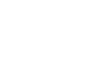to a quadrilateral K'L'M'N'.
1. Determine the coordinates of the image. (3 marks)
2. On the grid provided draw the object and the image. (2 marks)3.
1. Describe fully the transformation which maps KLMN onto K'L'M'N'.
2. Determine the area of the image.
4. Find a matrix which maps K'L'M'N' onto KLMN.
8. The first, fifth and seventh terms of an arithmetic progression (AP) correspond to the first three consecutive terms of a decreasing Geometric Progression (G.P.). The first term of each progression, is 64, the common difference of the AP is d and the common ratio of the G.P. is r.
1.
1. Write two equations involving d and r. (2 marks)
2. Find the values of d and r. (4 marks)
2. Find the sum of the first 10 terms of:
1. The arithmetic progression (A.P.); (2 marks)
2. The Geometric Progression (G.P.). (2 marks)

MARKING SCHEME

SECTION I (50 marks)

Answer all the questions from this section in the spaces provided.

1. Simplify (3 marks)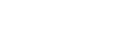2. By correcting each number to one significant figure, approximate the value of 788×0.006. Hence calculate the percentage error arising from this approximation. (3 marks)3. The area of triangle FGH is 21 cm3. The triangle FGH is transformed using the matrix.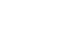Calculate the area of the image of triangle FGH (3 marks)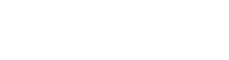4. Make s the subject of the formula. (3 marks)5. Solve the equation 2 log x — log (x—2) = 2 log 3. (3 marks)6. Kago deposited Ksh 30 000 in a financial institution that paid simple interest at the rate of 12% per annum. Nekesa deposited the same amount of money as Kago in another financial institution that paid compound interest. After 5 years, they had equal amounts of money in the financial institutions. Determine the compound interest rate, to 1 decimal place for Nekesa’s deposit. (4 marks)7. The masses in kilograms of 20 bags of maize were:
90, 94, 96, 98, 99, 102, 105, 91, 102, 99, 105, 94, 99, 90, 94, 99, 98, 96, 102 and 105.
Using an assumed mean of 96 kg, calculate the mean mass, per bag of the maize. (3 marks)8. The first term of an arithmetic sequence is —7 and the common difference is 3.
1. List the first six terms of the sequence;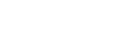2. Determine the sum of the first 50 terms of the sequence.9. A bag contains 2 white balls and 3 black balls. A second bag contains 3 white balls and 2 black balls. The balls are identical except for the colours. Two balls are drawn at random, one after the other from the first bag and placed in the second bag. Calculate the probability that the 2 balls are both white. (2 marks)10. An arc 11 cm long, subtends an angle of 70° at the centre of a circle. Calculate the length, correct to one decimal place, of a chord that subtends an angle of 90° at the centre of the same circle. (4 marks)

Length 12.7 cm

11. Given that qi + 1/3j + 2/3k is a unit vector, find q. (2 marks)12.
1. Expand the expression (1 + 1/2x)5 in ascending powers of x, leaving the coefficients as fractions in their simplest form. (2 marks)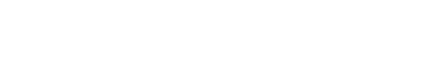2. Use the first three terms of the expansion in (a) above to estimate the value of (1 1/20)5. (2 marks)13. A circle whose equation is (x — l)2 + (y — k)2 = 10 passes through the point (2,5). Find the value of k. (3 marks)14. Water and milk are mixed such that the volume of water to that of milk is 4:1. Taking the density of water as 1 g/cm3 and that of milk as 1.2 g/cm3, find the mass in grams of 2.5 litres of the mixture. (3 marks)15. A school decided to buy at least 32 bags of maize and beans. The number of bags of beans were to be at least 6. A bag of maize costs Ksh 2 500 and a bag of beans costs Ksh 3 500. The school had Ksh 100 000 to purchase the maize and beans.
Write down all the inequalities that satisfy the above information. (4 marks)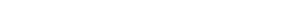16. Find in radians, the values of x in the interval 0o≤ x ≤2πfor which 2cos2x — sin x = 1. (Leave the answer in terms of π) (4 marks)SECTION II (50 marks)

Answer any five questions from this section in the spaces provided

1. A garden measures 10 m long and 8 m wide. A path of uniform width is made all round the garden. The total area of the garden and the path is 168 m2.
1. Find the width of the path. (4 marks)2. The path is to be covered with square concrete slabs. Each comer of the path is covered with a slab whose side is equal to the width of the path. The rest of the path is covered with slabs of side 50 cm. The cost of making each comer slab is Sh 600 while the cost of making each smaller slab is Sh 50.
Calculate:
1. the number of the smaller slabs used. (3 marks)2. the total cost of the slabs used to cover the whole path. (3 marks)2. In the figure below, P, Q, R and S are points on the circle with centre O. PRT and USTV are straight lines. Line USTV is a tangent to the circle at S. <RST = 50° and <RTV = 150°1. Calculate the size of:
1. <QRS; (2 marks)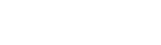2. <USP; (1 mark)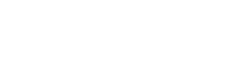3. <PQR. (2 marks)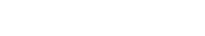2. Given that RT = 7 cm and ST = 9 cm, calculate to 3 significant figures;
1. the length of line PR; (2 marks)2. the radius of the circle. (3 marks)3. The figure ABCDEF below represents a roof of a house. AB = DC = 12m, BC = AD = 6m, AE = BF = CF = DE = 5m and EF = 8m.1. Calculate, correct to 2 decimal places, the perpendicular distance of EF from the plane ABCD. (4 marks)2. Calculate the angle between:
1. the planes ADE and ABCD; (2 marks)2. the line AE and the plane ABCD, correct to 1 decimal place; (2 marks)3. the planes ABFE and DCFE, correct to 1 decimal place. (2 marks)4. A water vendor has a tank of capacity 18 900 litres. The tank is being filled with water from two pipes A and B which are closed immediately when the tank is full. Water flows at the rate of 150 000cm3/minute through pipe A and 120 OOOcm3/minute through pipe B.
1. If the tank is empty and the two pipes are opened at the same time, calculate the time it takes to fill the tank. (3 marks)2. On a certain day the vendor opened the two pipes A and B to fill the empty tank. After 25 minutes he opened the outlet tap to supply water to his customers at an average rate of 20 litres per minute.
1. Calculate the time it took to fill the tank on that day. (4 marks)2. The vendor supplied a total of 542 jerricans, each containing 25 litres of water, on that day. If the water that remained in the tank was 6 300 litres, calculate, in litres, the amount of water that was wasted. (3 marks)5. A tourist took 1 hour 20 minutes to travel by an aircraft from town T(3°S, 35°E) to town U(9°N, 35°E). (Take the radius of the earth to be 6370km and π=22/7
1. Find the average speed of the aircraft. (3 marks)2. After staying at town U for 30 minutes, the tourist took a second aircraft to town V(9°N, 5°E). The average speed of the second aircraft was 90% that of the first aircraft. Determine the time, to the nearest minute, the aircraft took to travel from U to V. (3 marks)3. When the journey started at town T, the local time was 0700 h. Find the local time at V when the tourist arrived. (4 marks)6. The gradient function of a curve is given by the expression 2x + 1. If the curve passes through the point (—4, 6);
1. Find:
1. The equation of the curve, (3 marks)2. The values of x at which the curve cuts the x-axis, (3 marks)3. Determine the area enclosed by the curve and the x-axis. (4 marks)7. A quadrilateral with vertices at K(1,1), L(4,1), M(2,3) and M(1,3) is transformed by a matrix.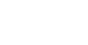1. Determine the coordinates of the image. (3 marks)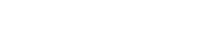2. On the grid provided draw the object and the image. (2 marks)3.
1. Describe fully the transformation which maps KLMN onto K'L'M'N'.
Shear parallel to x-axis of shear factor 3 with the x-axis as the invariant
2. Determine the area of the image.
Area of triangle= Area of object=4 sq units
4. Find a matrix which maps K'L'M'N' onto KLMN.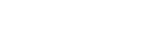8. The first, fifth and seventh terms of an arithmetic progression (AP) correspond to the first three consecutive terms of a decreasing Geometric Progression (G.P.). The first term of each progression, is 64, the common difference of the AP is d and the common ratio of the G.P. is r.
1.
1. Write two equations involving d and r. (2 marks)
64+4d=64r
64+6d=64r2
2. Find the values of d and r. (4 marks)2. Find the sum of the first 10 terms of:
1. The arithmetic progression (A.P.); (2 marks)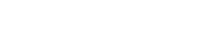2. The Geometric Progression (G.P.). (2 marks)• ✔ To read offline at any time.
• ✔ To Print at your convenience
• ✔ Share Easily with Friends / Students